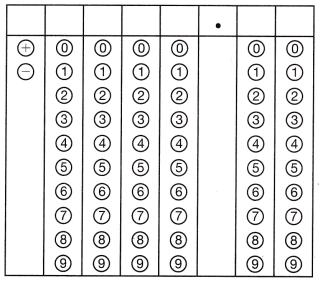Refer to our Texas Go Math Grade 8 Answer Key Pdf to score good marks in the exams. Test yourself by practicing the problems from Texas Go Math Grade 8 Module 6 Quiz Answer Key.

6.1 Identifying and Representing Functions

Determine whether each relationship is a function.

Question 1.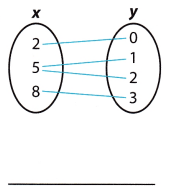Not a function A relationship is a function when each input is paired with exactly one output. The input 5 has more than one output

Question 2.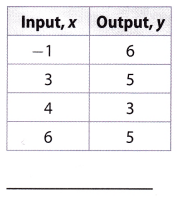To determine the answer here, we will review the definition of a function.

A certain relationship represents a function if every input is paired with only one output. Considering the fact that in a given table every input has only one output we can conclude that it represents a function.

Question 3.
(2, 5), (7, 2), (-3, 4), (2, 9), (1, 1)
Not a function A relationship is a function when each input is paired with exactly one output. The input 2 has more than one output

6.2 Describing Functions

Determine whether each situation is linear or nonlinear, and proportional or nonproportional.

Question 4.
Joanna is paid $14 per hour. Answer: To determine if the situation is linear or nonlinear. Let us analyze if we can express the situation in linear equation. Given the situation Joana is paid$14 per hour, we can express this in linear equation using the slope-intercept from y = mx + b.
Let y be the amount to be paid to Joana x be the number of hours m is the rate of payment per hour

The equation that can be formed is y = 4x

Since, the equation formed is linear equation, then the situation is linear.

To determine if the given situation is proportional or nonproportional, let us have the equation y = 4x and determine the value of b. If b = 0, then the equation is proportional and if b ≠ 0, then the equation is nonproportional
Since the equation of the given situation is y = 4x, where b = 0, then the equation is proportional.
Thus, the situation is proportional.
linear; proportional

Question 5.
Alberto started out bench pressing 50 pounds. He then added 5 pounds every week.
To determine if the situation is linear or nonlinear. Let us analyze if we can express the situation in linear equation.

Given the problem “Alberto started out bench pressing 50 pounds. He then added 5 pounds every week”. We can
express this in linear equation using the slope-intercept form y = mx + b.
Let y be the number of Alberto’s bench pressing in pounds
x be the number of weeks
m be the additional pounds every week
b be the number of pounds when Alberto started bench pressing

The equation that can be formed where m = 5 and b = 50 is
y = mx + b
y = 5x + 50
Since, the equation formed is linear equation, then the situation is linear.
To determine if the given situation is proportional or nonproportional, let us have the equation and determine the
value of b. If b = 0, then the equation is proportional if b ≠ 0, then the equation is nonproportional.

Since the equation of the given situation is y = 5x + 50, where b ≠ 0, then the equation is nonproportional.
Thus, the situation is nonproportional.
linear; nonproportional

6.3 Comparing Functions

Question 6.
Which function is changing more quickly? Explain.To determine the answer here, we will calculate the average rate of change for given functions. We will calculate
the rate using following formula: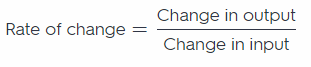Therefore, we can calculate the average rate of change for function 1 as following:
Rate of change = $$\frac{0-20}{5-0}$$
= -4

Let’s calculate the rate of change for function 2:
\begin{align*}
\text{ Rate of change}&=\dfrac{2-11}{4-2}\\
&=-4.5
\end{align}

To determine which function is changing more quickly, we have to compare the absolute values of obtained
numbers. Considering the fact that:
|-4| < |-4.5|,
we can conclude that function 2 changes more quickly.

Essential Question

Question 7.
How can you use functions to solve real-world problems?
The situation gives a graph that is a straight line which is linear since it is stated that Sam is running at a constant
rate, meaning the distance covered per unit time always of the same value.

Another thing is that, since the distance covered per unit time always of the same value, then the graph has a
proportional relationship that pass through the origin.

See the explanation.

Texas Go Math Grade 8 Module 6 Mixed Review Texas Test Prep Answer Key

Selected Response

Question 1.
Which table shows a proportional function?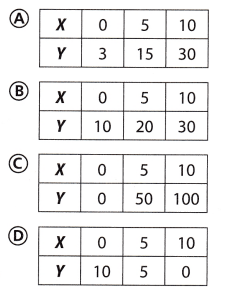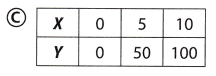Explanation:
Option C represents a proportional relationship. It contains the ordered pair of the origin (0, 0)

Question 2.
Which term does not correctly describe the relationship shown in the table?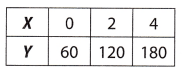(A) function
(B) linear
(C) proportional
(D) nonproportional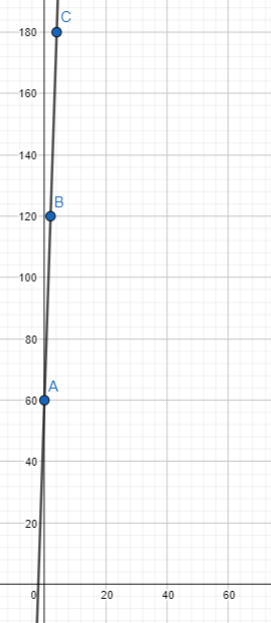Option B is the correct answer.

Question 3.
The table below shows some input and output values of a function.What is the missing output value?
(A) 20
(B) 21
(C) 22
(D) 23
17.5 – 14 = 3.5
17.5 + 3.5 = 21Option B is the correct answer.

Question 4.
Which number is not rational?
(A) -7
(B) $$\sqrt{25}$$
(C) 0.33
(D) $$\sqrt{15}$$
(A) -7 is a rational number because 7 corresponds to the definition of a rational number.
(B) $$\sqrt{25}$$ is a rational number.
(C) 0.33 is a rational number.
(D) $$\sqrt{15}$$ The square root of 15 is not a rational number. It is an irrational number.

Question 5.
Which of the relationships below is a function?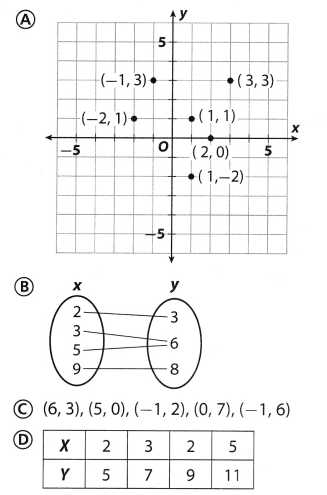Gridded Response

Question 6.
A dance school charges a registration fee in addition to a fee per lesson. The equation y = 30x + 25 represents the total cost y of x lessons. What is the cost per lesson?